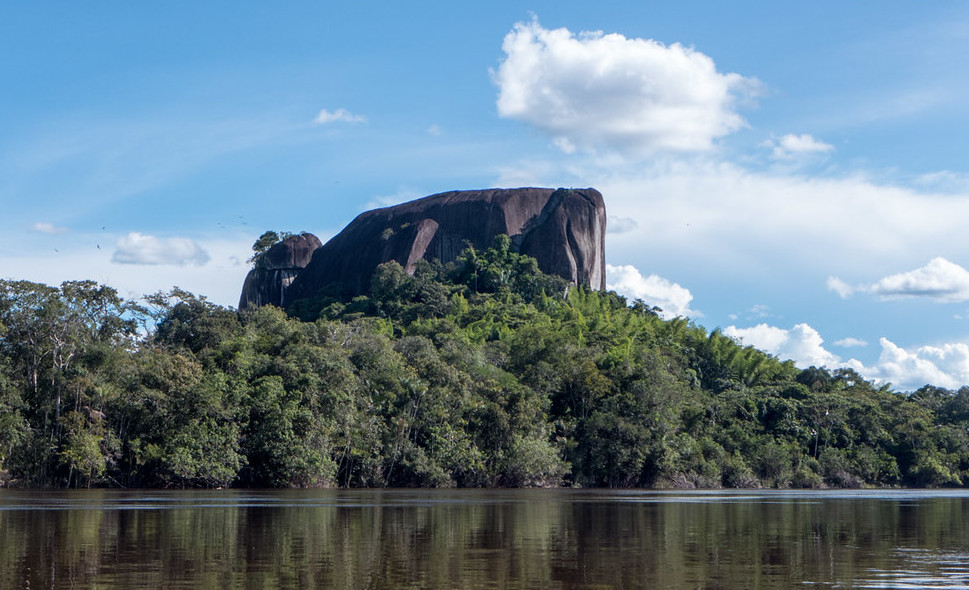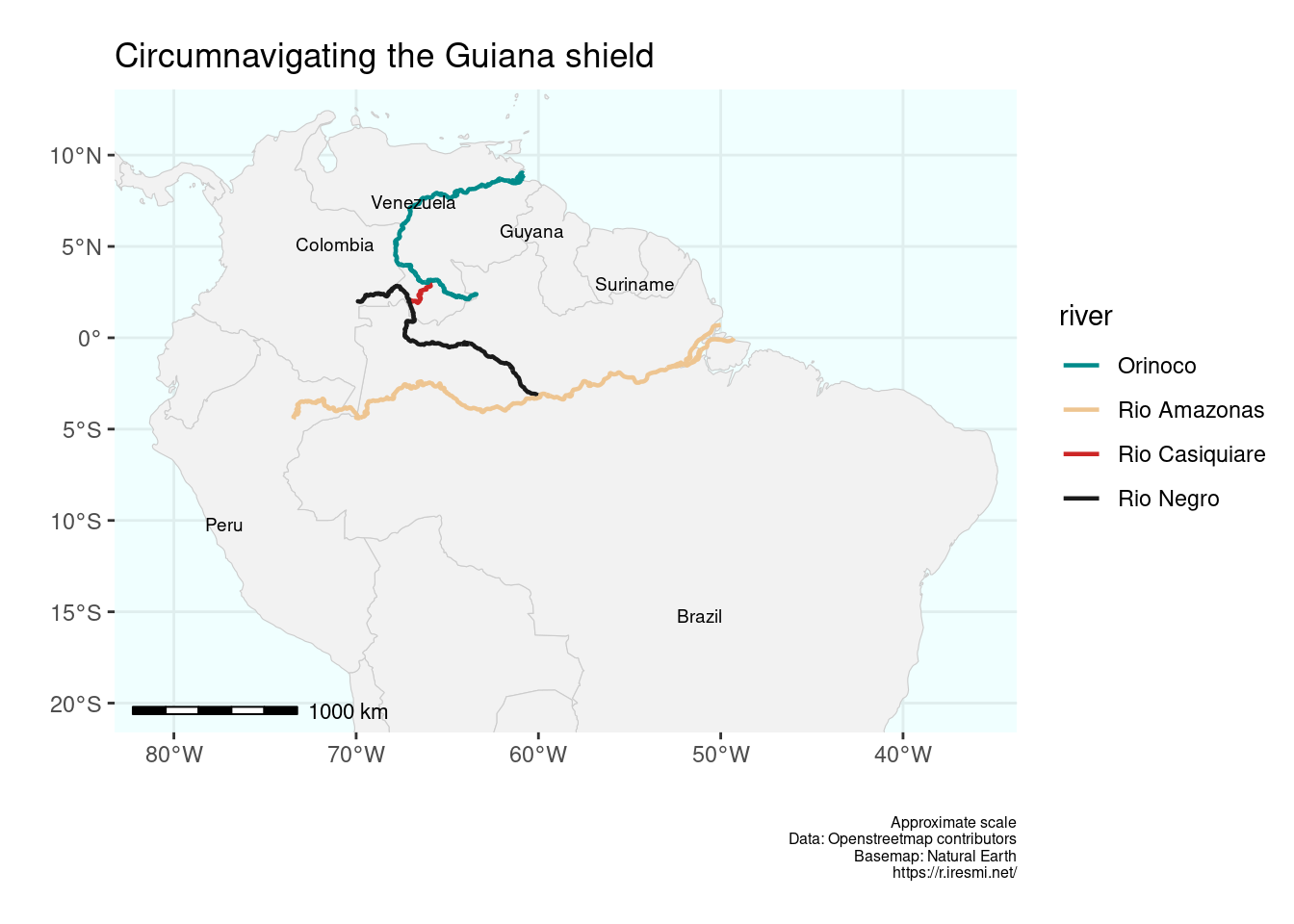# Casiquiare

Day 12 of 30DayMapChallenge
R
30DayMapChallenge
spatial
datavisualization
OSM
Author

Michaël

Published

2023-11-12

Modified

2023-11-11Piedra de Culimacare on the Rio Casiquiare – CC-BY-NC-ND by Carsten ten Brink

Day 12 of 30DayMapChallenge: « South America » (previously).

Do you know you can circumnavigate the Guiana shield? There is a river, the Rio Casiquiare, that connects the Orinoco and the Amazon basin! If you need a map for your next adventure, in the “footsteps” of Alexander von Humboldt, here it is…

We pick the OpenStreetMap ID of the river relations (e.g.: https://www.openstreetmap.org/relation/2295651), make a `data.frame` of the rivers geometry and plot it.

``````library(tidyverse)
library(sf)
library(rnaturalearth)
library(osmdata)
library(ggspatial)
library(ggrepel)

countries <- ne_countries(scale = 50, returnclass = "sf")

rivers <- tribble(~name,            ~relation,
"Rio Casiquiare", 275909,
"Orinoco",        1083284,
"Rio Negro",      275660,
"Rio Amazonas",   2295651) |>
mutate(geometry = map(relation, \(r) paste0("relation/", r) |>
opq_osm_id() |>
opq_string () |>
osmdata_sf () |>
pluck("osm_lines", "geometry") |>
st_union() |>
st_geometrycollection())) |>
st_sf(crs = "EPSG:4326")``````
``````ggplot() +
geom_sf(data = countries, color = "gray80", fill = "gray95") +
geom_sf(data = rivers, aes(color = name), linewidth = .8, key_glyph = "smooth") +
geom_text_repel(data = countries |>
filter(adm0_a3 %in% c("BRA", "GUY", "VEN", "SUR", "COL", "PER")),
aes(label = name, geometry = geometry),
stat = "sf_coordinates", size = 2.5) +
scale_color_manual(name = "river",
values = c("Rio Casiquiare" = "firebrick3",
"Orinoco"        = "cyan4",
"Rio Negro"      = "gray10",
"Rio Amazonas"   = "burlywood2")) +
coord_sf(xlim = c(-81, -36), ylim = c(-20, 12)) +
labs(title = "Circumnavigating the Guiana shield",
caption = "Approximate scale\nData: Openstreetmap contributors\nBasemap: Natural Earth\nhttps://r.iresmi.net/",
x = "",
y = "") +
annotation_scale(height = unit(1, "mm")) +
theme(panel.background = element_rect(fill = "azure"),
panel.grid = element_line(color = "azure2"),
legend.key = element_rect(fill = NA),
plot.caption = element_text(size = 6))``````